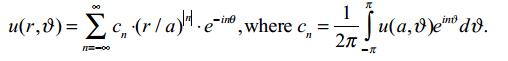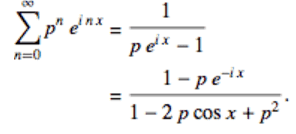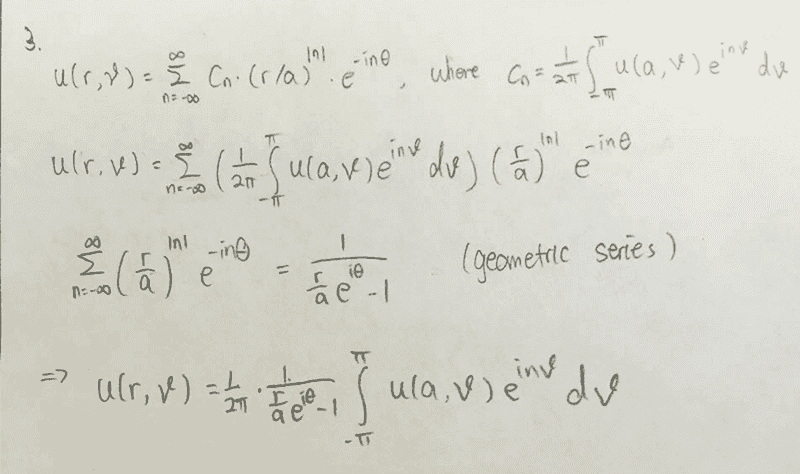# Heat conduction problem in a ring of radius a

• rx24race

## Homework Statement

We previously solved the heat conduction problem in a ring of radius a, and the solution isc into the sum, perform the sum first (which is just a geometric series), and obtain the general solution, which should only involve one integral in ϑ

## Homework Equations## The Attempt at a Solution[/B]

where is this geometric series come from Never seen it before